# I.E. Irodov Solutions on Transport Phenomena

Transport Phenomena chapter 2.7 solved problems covered in I.E. Irodov textbook available here. In Physics, transport phenomenon is any of the phenomena involving the movement of various entities, like momentum, mass, energy, through a medium, fluid or solid, by virtue of nonuniform conditions existing within the medium. The questions asked in I.E. Irodov textbook Chapter 2.7 are at a standard level for the students preparing for competitive exams. Solving these types of problems builds the confidence of students and enables them to have a grip over the subject. Students can access I.E. Irodov book PART TWO. THERMODYNAMICS AND MOLECULAR PHYSICS - 2.7 Transport Phenomena solutions at BYJU’S.

### I.E. Irodov Solutions on Transport Phenomena

1. Calculate what fraction of gas molecules

1. (a) traverses without collisions the distances exceeding the mean free path λ;

2. (b) has the free path values lying within the interval from λ to 2λ.

Solution:

1. (a) The fraction of gas molecules which traverses distances exceeding the mean free path without collision is just the probability to traverse the distance s = λ without collision.

Therefore, P = 1/e = 0.37

(b) This probability is, P = 1/e - 1/e2 = 0.23

2. A narrow molecular beam makes its way into a vessel filled with gas under low pressure. Find the mean free path of molecules if the beam intensity decreases η-fold over the distance Δl.

Solution:

1. From the formula,

1/η = 1/e∆l/λ or λ = ∆l/ln η

3. Let α dt be the probability of a gas molecule experiencing a collision during the time interval dt; α is a constant. Find:

1. (a) the probability of a molecule experiencing no collisions during the time interval t;

2. (b) the mean time interval between successive collisions.

Solution:

1. (a) Let P (f) = probability of no collision in the interval (0, t). Then,

p(t + dt) = p(t)(1 - αdt)

or dP/dt = -α p(t) or p(t)= 1/eαt

Where we have used P (0) = 1

(b) The mean interval between collisions is also the mean interval of no collision. Then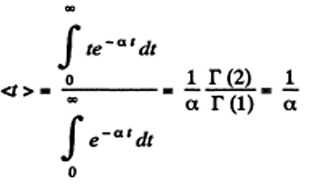4. Find the mean free path and the mean time interval between successive collisions of gaseous nitrogen molecules

1. (a) under standard conditions;

2. (b) at temperature t = 0°C and pressure p = 1.0 nPa (such a pressure can be reached by means of contemporary vacuum pumps)

Solution:

1.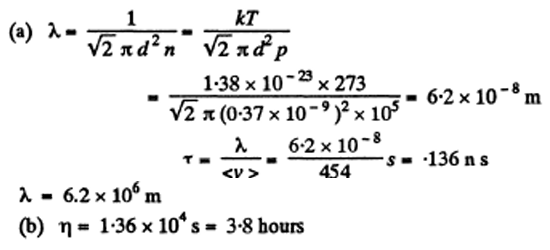5. How many times does the mean free path of nitrogen molecules exceed the mean distance between the molecules under standard conditions?

Solution:

1. The mean distance between molecules is of the order

([22.4 x 10-3]/[6x1023])1/3 = 3.34 x 10-9 mtrs

This is about 18.5 times smaller than the mean free path.

6. Find the mean free path of gas molecules under standard conditions if the Van der Waals constant of this gas is equal to b = 40 ml/mol.

Solution:

1. The Vander Waal’s constant b is four times the molecular volume,

b = 4 NA(π/6)d3 or d = (3b/2 πNA)1/3

So, λ = (kTo/ √2πpo)(2πNA/3b)2/3

7. An acoustic wave propagates through nitrogen under standard conditions. At what frequency will the wavelength be equal to the mean free path of the gas molecules?

Solution:

1. The velocity of sound in N2 is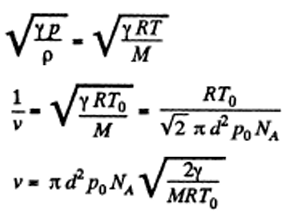8. Oxygen is enclosed at the temperature 0°C in a vessel with the characteristic dimension l = 10 mm (this is the linear dimension determining the character of a physical process in question). Find:

1. (a) the gas pressure below which the mean free path of the molecules λ > 1;

2. (b) the corresponding molecular concentration and the mean distance between the molecules.

Solution:

1. (a) λ > l if p < kT/√2𝜋d2l

Now, kT/√2𝜋d2l for O2 of O is 0.7 Pa.

(b) The corresponding n is obtained by dividing by kT and is 1.84x1020 per m3 = 1.84 upper c.c. and the corresponding mean distance is l/nl/3.

= 10-2/[0.1841/3 x 105] = 0.18 μm.

9. For the case of nitrogen under standard conditions find:

1. (a) the mean number of collisions experienced by each molecule per second;

2. (b) the total number of collisions occurring between the molecules within 1 cm3 of nitrogen per second

Solution:

1. (a) v = 1/𝜏 = 1/λ/<v> = <v>/λ

= √2𝜋d2n<v> = .74 x 1010 s-1

(b) Total number of collisions is

(1/2) nv = 1.0 x 1029 s cm-3

Note, the factor 1/2. s within 1 cm When two molecules collide we must not count it twice.

10. How does the mean free path λ and the number of collisions of each molecule per unit time v depend on the absolute temperature of an ideal gas undergoing (a) an isochoric process; (b) an isobaric process?

Solution:

1. (a) λ = 1/√2𝜋d2n

d is a constant and n is a constant for an isochoric process so X is constant for an isochoric process.

v = <v>/ λ = [(√8RT/M𝜋)/λ ] α √T

(b) λ = 1/(√2 𝜋 d2) x (KT/p) α √T, for an isobaric process.

v = <v>/ λ α √T/T = 1/√T , an isobaric process.

11. As a result of some process the pressure of an ideal gas increases n-fold. How many times have the mean free path λ and the number of collisions of each molecule per unit time v changed and how, if the process is (a) isochoric; (b) isothermal?

Solution:

1. (a) In an isochoric process λ is constant and

v α √T α √pV α √p α √n

(b) λ = KT/(√2 𝜋 pd2) must decrease n times in an isothermal process and v must increase n times because <v> is constant in an isothermal process.

12. An ideal gas consisting of rigid diatomic molecules goes through an adiabatic process. How do the mean free path λ and the number of collisions of each molecule per second v depend in this process on (a) the volume V; (b) the pressure p; (c) the temperature T?

Solution:

1. λ α 1/n

Or 1/N/V = V/N

Therefore, λ α V and v α T1/2/V

But in an adiabatic process, γ = 7/5

TV γ-1 = constant so TV2/5 = constant

Or T1/2 α V-1/5. Thus, v α V-6/5.

(b) λ α T/p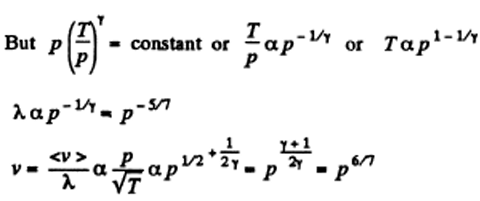(c) λ α V

But TV2/5 = constant or λ α T-5/2

Thus, v α T1/2/V α T3

13. An ideal gas goes through a polytrophic process with exponent n. Find the mean free path and the number of collisions of each molecule per second v as a function of (a) the volume V; (b) the pressure p; (c) the temperature T.

Solution:

1. In the polytrophic process of index n

pVn = constant TVn-1= constant and p1-nTn= constant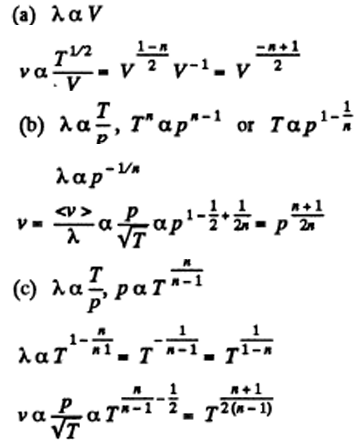14. Determine the molar heat capacity of a polytropic process through which an ideal gas consisting of rigid diatomic molecules goes and in which the number of collisions between the molecules remains constant (a) in a unit volume; (b) in the total volume of the gas.

Solution:

1. The number of collisions between the molecules in a unit volume is

(1/2)nv = (1/√2) 𝜋d2n2 <v> α √T/V2

This remains constant in the poly process pV-3 = constant

We know, the molar specific heat for the polytrophic process

pVα = constant

C = R[1/( γ-1) - 1/( α-1)]

C = R[1/( γ-1) + 1/4] = (11/4) R

Above equation also be written as (1/4)R(1+2i) where i= 5.

(b) √T/V = constant and pV-1= constant

So, C = R[1/( γ-1) + 1/2] = 3 R

Above equation also be written as (R/2) (i+1).

15. An ideal gas of molar mass M is enclosed in a vessel of volume V whose thin walls are kept at a constant temperature T. At a moment t = 0 a small hole of area S is opened, and the gas starts escaping into vacuum. Find the gas concentration n as a function of time t if at the initial moment n (0) = n0.

Solution:

1. We can assume that all molecules, incident on the hole, leak out. Then,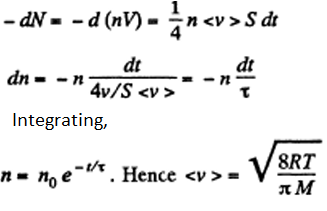16. A vessel filled with gas is divided into two equal parts 1 and 2 by a thin heat-insulating partition with two holes. One hole has a small diameter, and the other has a very large diameter (in comparison with the mean free path of molecules). In part 2 the gas is kept at a temperature η times higher than that of part 1. How will the concentration of molecules in part 2 change and how many times after the large hole is closed?

Solution:

1. If the temperature of the compartment 2 is η times more than that of compartment 1, it must contain 1/η times less number of molecules since pressure must be the same when the big hole is open. If M - mass of the gas in 1 than the mass of the gas in 2 must be M/η. So immediately after the big hole is closed.

n1o = M/mV, n20 = M/mVη

Where m = mass of each molecule and n10, n20 are concentrations in 1 and 2. After the big hole is closed the pressures will differ and concentration will become n1 and n2 where

n1 +n2 = [M/ mVη] (1+η)

on other hand,

n1<v1> = n2<v2> i.e. n1 = √ηn2

n2 (1+√η) = [m/mVη] (1+η) = n20 (1+η)

so, n2 = n20 [(1+η)/(1++√η)]

17. As a result of a certain process the viscosity coefficient of an ideal gas increases α = 2.0 times and its diffusion coefficient β = 4.0 times. How does the gas pressure change and how many times?

Solution:

1. We know, η = (1/3)<v> ρλ = (1/3)<v> 1/[ √2 𝜋d2]m α√T

Therefore, η changing α times implies T changing α2 times. On the other hand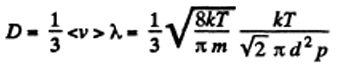Thus D changing P times means T3/2/p changing β times. So p must change α3/β times.

18. How will a diffusion coefficient D and the viscosity coefficient η of an ideal gas change if its volume increases n times: (a) isothermally; (b) isobarically?

Solution:

1. D α √T/n α V√T, η α √T

(a) D will increase n times

η will remain constant if T is constant

(b)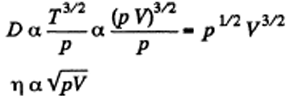Therefore, D will increase n3/2 times, η will increase n1/2, if p is constant.

19. An ideal gas consists of rigid diatomic molecules. How will a diffusion coefficient D and viscosity coefficient η change and how many times if the gas volume is decreased adiabatically n =10 times?

Solution:

1. D α V√T, η α T

In an adiabatic process, TVγ-1 = constant or T α Vγ-1

Now V is decreased 1/n times.

D α V(3-γ)/2 = (1/n) (3-γ)/2 = (1/n)4/5

η α of V(1-γ)/2 = (n)1/5

So D decreases n4/5 times and η increase n1/5 times.

20. An ideal gas goes through a polytrophic process. Find the polytrophic exponent n if in this process the coefficient (a) of diffusion; (b) of viscosity; (c) of heat conductivity remains constant.

Solution:

1. D α V√T α √(pV3)

D remains constant in the process pV3 = constant

So polytrophic index n = 3

(b) η α √T α √pV

η remains constant in the isothermal process.

pV = constant, n = 1, here

(c) Heat conductivity k = ηCv and Cv is a constant for the ideal gas.

Hence, n = 1 here also.